Courses

# Compass and Traverse Surveying Civil Engineering (CE) Notes | EduRev

## Civil Engineering (CE) : Compass and Traverse Surveying Civil Engineering (CE) Notes | EduRev

The document Compass and Traverse Surveying Civil Engineering (CE) Notes | EduRev is a part of the Civil Engineering (CE) Course Geomatics Engineering (Surveying).
All you need of Civil Engineering (CE) at this link: Civil Engineering (CE)

Question 1: The data from a closed traverse survey PQRS (run in the clockwise direction) are given in the table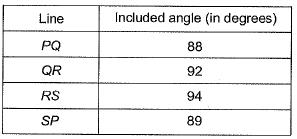The closing error for the traverse PQRS (in degrees) is ________.    [2019 : 1 Mark, Set-II]

Solution: Assuming it as anticlockwise traverse. Mathematically sum of interior angle for a closed traverse
= (2n - 4) x 90
= (2 x 4 - 4) x 90
= 4 x 90 = 360°
Given sum of interior angles, = 88 + 92 + 94 + 89 = 363°
Then error in interior angle = 363 - 360 = 3°
Note: In this question as per clockwise traverse included angle should be taken as exterior angle. But if we take exterior angle then we get all interior angles more than 180°.

Question 2: The interior angles of four triangles are given below: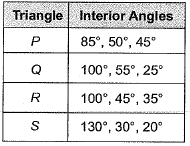Which of the triangles are ill-conditioned and should be avoided in Triangulation surveys?    [2019 : 1 Mark, Set-I]
(a) Both Q and S
(b) Both P and S
(c) Both Q and R
(d) Both P and R
Solution: For a well conditioned triangle.
The interior angle should not be less than 30°. In this way, triangle ‘Q’ & S having less angles (acute angle).
⇒ Q & S are ill-conditioned.

Question 3: The following details refer to a closed traverse: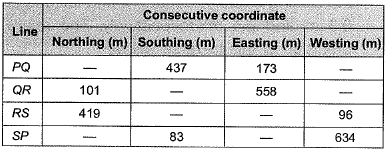The length and direction (whole circle bearing) of closure, respectively are    [2018 : 2 Marks, Set-I]
(a) 1 m and 90°
(b) 2 m and 90°
(c) 1 m and 270°
(d) 2 m and 270°
Solution:
∑L = W northing- E southing
= (101 + 419) - (437 + 83)
= 0
∑D = E Easting - E Westing
= (173 + 558) - (96 + 634)
= 1 m
∴ Length of closure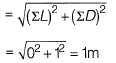And, direction of closure,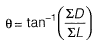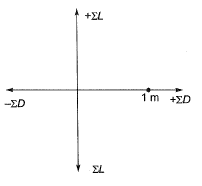Hence, θ lies in I quadrant and θ = 90°

Question 4: The observed bearings of a traverse are given below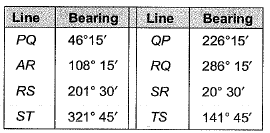The station(s) most likely to be affected by the local attraction is/are     [2017 : 2 Marks, Set-I]
(a) Only R
(b) Only S
(c) Rand S
(d) Pand Q
Solution: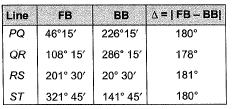Station R is most likely to be affected.
Since difference of FB and BB for PQ and ST is 180°.
Hence, P, Q, S, T are free from local attraction.

Question 5: The reduced bearing of a 10 m long line is N30°E. The departure of the line is    [2016 : 1 Mark, Set-II]
(a) 10.00 m
(b) 8.66 m
(c) 7.52 m
(d) 5.00 m
Solution: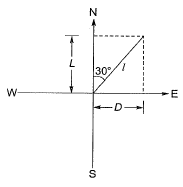The departure of the line,
D = l sin θ
= 10 sin 30°
= 10/2 = 5m

Question 6: The bearings of two inaccessible stations, S1 (Easting 500 m, Northing 500 m) and S2 (Easting 600 m, Northing 450 m) from a station S3 were observed as 225° and 153° 26' respectively. The independent Easting (in m) of station S3 is:    [2015 : 2 Marks, Set-II]
(a) 450.000
(b) 570.710
(c) 550.000
(d) 650.000
Solution: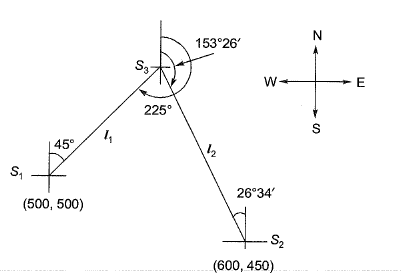Let S1S3 = l1 and S2S3 = l2
Northing of S3:
500 + l1cos45°= 450 + l2cos26°34'
l1 cos 45° - l2cos 26° 34' = - 50    ..(i)
Easting of S3
500 + l1 sin 45° = 600 - l2sin26°34'
l1sin45° + l2sin26°34' = 100     ..(ii)
Solving eq. (i) and eq. (ii),
l= 70.707
l2 = 111.802
∴ Easting of S3 = 500 + 70.707 sin45°
= 550m

Question 7: In a region with magnetic declination of 2°E, the magnetic Fore Bearing (FB) of a line AB was measured as N79°50'E. There was local attraction at A. To determine the correct magnetic bearing of the line, a point 0 was selected at which there was no local attraction. The magnetic FB of line AO and OA were observed to be S52°40'E and N50°20'W, respectively. What is the true FB of line AB?    [2015 : 2 Marks, Set-I]
(a) N81°50'E
(b) N82°10'E
(c) N84°10'E
(d) N77°50'E
Solution: Magnetic Declination,δ = 2°E
Magnetic FB of AB = N 79°50' E
To find local attraction at station A
As station O is free from local attraction
Hence FB of OA will be correct,
Correct FB of OA = N 50°20' W ≌ 309°40'
∴ Correct BB of OA = 129°40'
∵ Obserbed FB of AO
= Observed BB of OA
= S52°40'E
= 127°20
Error = Observed bearing - Correct bearing
= 127°20' - 129°40'
= 2°20'
Correction = +2°20'
Local attraction at station A
= +2°20' ≌ 2°20' E
∴ Magnetic FB of AB = N 79°50'E
δ = 2°E and local attraction = 2°20'E
∴ TB of FB of AB = 79°50' + 2°20' + 2°
= N84°10'E

Question 8: In a closed loop traverse of 1 km total length, the closing errors in departure and latitude are 0.3 m and 0.4 m, respectively. The relative precision of this traverse will be    [2015 : 1 Mark, Set-I]
(a) 1 : 5000
(b) 1 : 4000
(c) 1 : 3000
(d) 1 : 2000
Solution: Relative precision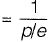where, p = Perimeter of traverse
e = Closing error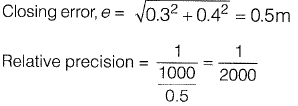Question 9: Group-I lists tool/instrument while Group-ll lists the method of surveying. Match the tool/ instrument with the corresponding method of surveying.    [2014 : 2 Marks, Set-I]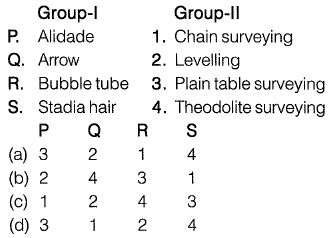Solution: 1. Alllade: It is a straight edge ruler used in plane table surveying whichis used for sighting the objects and drawing the line son the drawing sheet.
2. Arrow: Arrows are used to mark the position of end of the hain or tape on the ground.
3. Bubble tube: It is used to check the level of the instrument. When the bubble of the tube comes in the centre, then the instrument in levelled.
4. Stadia hair: Lines on diaphragm, the intercept ‘S’ between 2 stadia hairs of a vertically held rod gives the distance between tacheometer and the road as
D = kS (k = stadia constant)

Question 10: A Theodolite is placed at A and a 3 m long vertical staff is held at B. The depression angle made at reading of 2.5 m marking on staff is 6°10'. The horizontal distance between A and B is 2200 m. The height of instrument at A is 1.1 m and reduced level of point A is 880.88 m using curvature correction and refraction correction determine the R.L. of point B(in m)?    [2013 : 2 Marks]
Solution: RL of A = 880.88 m
RL of plane of collimation
= 880.88 + 1.1 = 881.98 m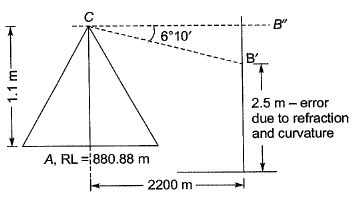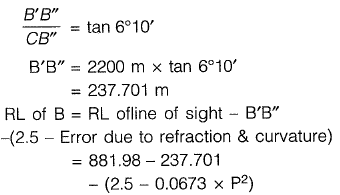Here, D = Distance between theodolite and staff in km
∴ RL of B = 881.98-237.701
- (2.5 x 0.0673 x 2.22)
= 642.105 m

Question 11: Bearing of the given system is shown below: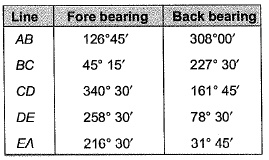Applying correction due to local attraction, the correct bearing of line BC will be    [2013 : 2 Marks]
(a) 48°15'
(b) 50° 15'
(C) 49° 15'
(d) 48°45'
Solution: The difference between back bearing and fore bearing of line DE
= 258° 30' - 78° 30' = 180°
∴ Station D and E are free from local attraction
Fore bearing of line EA = 216° 30'
∴ Correct back bearing of line EA
= 216° 30' - 180° = 36° 30'
∴ Error at A = 31° 45' - 36° 30' = -4°45'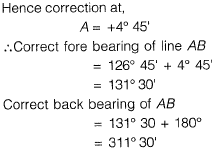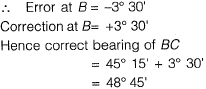Question 12: The latitude and departure of a line AB are +78 m and -45.1 m respectively. The whole circle bearing of the line AB is    [2013 : 1 Mark]
(a) 30°
(b) 150°
(c) 210°
(d) 330°
Solution: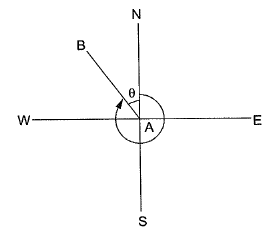Since the latitude of line is positive and departure is negative, the line lies in the fourth quadrant.
∴ L*cosθ = 78
L*sinθ = -45.1
⇒ tanθ = -0.578
θ = -30°
∴ WCB of AB = 360° - 30°
= 330°

Question 13: The observations from a closed loop traverse around an obstacle are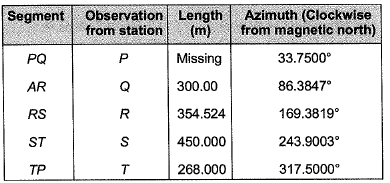What is the value of the missing measurement (rounded off to the nearest 10 mm)?    [2011 : 2 Marks]
(a) 396.86 m
(b) 396.79 m
(c) 396.05 m
(d) 396.94 m

Solution: In a closed loop travers, the algebraic sum of all the latitudes should be equal to zeroi.e., ∑L = 0
⇒ Lcos 33.7500°+ 300 cos 86.3847° + 354.524 cos 169.3819° + 450 cos 243.900° + 268 cos 317.5° = 0
⇒ Lcos 33.75° = 329.9166
⇒ L = 396.79 m

Offer running on EduRev: Apply code STAYHOME200 to get INR 200 off on our premium plan EduRev Infinity!

## Geomatics Engineering (Surveying)

19 videos|15 docs|17 tests

,

,

,

,

,

,

,

,

,

,

,

,

,

,

,

,

,

,

,

,

,

;